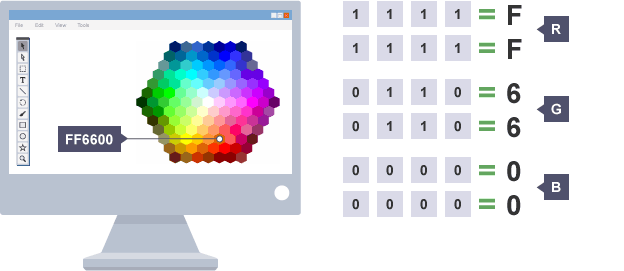home

# What the hex is Hexadecimal?!

Hexidecimal is a way of representing numbers using the base 16 instead of base 10 (decimal) which we are used to! Hexadecimal would likely be completely normal to us if we had 16 fingers instead of 10.It is very common to see in computing or programming, as often colours, memory addresses and binary are represented using it. This is because hexadecimal is a fairly easy way of representing binary numbers (I will explain this more later!).

There are 16 different hexadecimal digits: 1, 2, 3, 4, 5, 6, 7, 8, 9, A, B, C, D, E and F. They can be translated to decimal numbers very easily:

0 0
1 1
2 2
3 3
4 4
5 5
6 6
7 7
8 8
9 9
A 10
B 11
C 12
D 13
E 14
F 15

When you are counting using the decimal numerical system, you are actually counting using base 10 without thinking about it. This means that every decimal numerical representation is based on 10:For example:

1 100 160
10 101 10 × 160
16 101 + (6 × 100 ) 161
100 102 (6 × 161) + (4 × 160)
3 3 × 100 3 × 160
35 (3 × 101) + (5 × 100) (2 × 161) + (4 × 160)

As I mentioned previously, Hexadecimal is used very often for representing binary! You will often see it used to represent colours (e.g. `#FFFFFF` = white) and memory addresses (e.g. `0xFFF`).Source: BBC
Hexadecimal is used for these purposes as you can easily encode binary as hexadecimal, by grouping 4 binary digits (bits) into 1 hexadecimal digit. For example, `1111` equals 15 in decimal, so can just be written as `F` in hexadecimal. You can see how this means you can represent binary in much fewer characters.